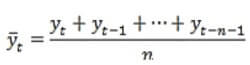# What is the moving average SPI?

For SPI-n you should calculate average of last n month for each month. In fact, the SPI-n is monthly that each month of rainfall is equal to average of last n month for each month. For example you want to calculate 12-month moving average of Mar-2000, you should calculate average of monthly rainfall from Apr-1999 to Mar-2000 and for calculating 12-month moving average of Apr-2000, then calculate average of monthly rainfall from May-1999 to Apr-2000. After the process done, you will have 12 number of rainfall for each year and you should calculate SPI with these data.Simple Moving Average Formula

Name: Hidden